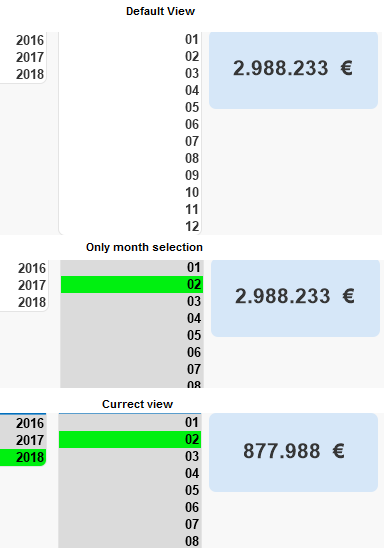# QlikView App Dev

Discussion Board for collaboration related to QlikView App Development.

Announcements
Customer & Partners, DEC. 9, 11 AM ET: Qlik Product & Strategy Roadmap Session: Data Analytics REGISTER NOW
cancel
Showing results for
Did you mean:Partner

## Performance and clearness

Hello community,

I've tried to implement a very useful formula within my qlikview application and was very suprised about the impact on the application-performance.

If(GetSelectedCount(BILL_MONTH) = 0,

SUM({1<DATATYPE={'ACTUAL'},BILL_YEAR = {\$(=If(GetSelectedCount(BILL_YEAR) = 0, date(addmonths(today(),-1),'YYYY'), Concat(BILL_YEAR, ',')))},BILL_MONTH={\$(=Concat(BILL_MONTH, ','))}>}SALES)

)

The purpose of this code snippet is to provide the user a default view if no selection was made. GetSelectedCount is a very useful function but it made the code a bit more complex. E.g. the main reason of the surrounding if-condition is, that I'm not able to tell qlik that it should take all months less than month today.

BILL_MONTH = {\$(=If(GetSelectedCount(BILL_MONTH) = 0, date(addmonths(today(),-1),'YYYY'), Concat(BILL_MONTH, ',')))}

What I need here is a less-than-statement ('<='). I didn't figure it out yet. Maybe u guys have an idea or a less complex proposal than mine.

3 RepliesMVP & Luminary

I think you could use something like this:

SUM({1<

DATATYPE={'ACTUAL'},

BILL_YEAR = \$(=pick(ceil(getselectedcount(BILL_YEAR) / 100) + 1,

'{"<' & num(month(today())) & '"}', 'p(BILL_YEAR)')),

BILL_MONTH = \$(=pick(ceil(getselectedcount(BILL_MONTH) / 12) + 1,

'{"<' & num(month(today())) & '"}', 'p(BILL_MONTH)'))

>} SALES)

which avoids the doubled calculation through the if-loop (all branches of an if-loop will always be calculated) and further the concat-aggregations within set analysis which without a DISTINCT might return a very large data-set.

- MarcusPartner
Author

Hi Marcus,

your approach is pretty good regarding to the performance. We need to change somenthing little to make it perfect.

The requirement is if the use doesn't make any selections:

BILL_YEAR = year(today())

BILL_MONTH < month(today())

So far, so good.

Just assuming the user selects last year the function is still showing <month(today()) but for the selected year (e.g. last year).

I tried to go with this:

SUM({1<

DATATYPE={'ACTUAL'},

BILL_YEAR = \$(=pick(ceil(getselectedcount(BILL_YEAR) / 100) + 1,

'{"' & year(today()) & '"}', 'p(BILL_YEAR)')),

BILL_MONTH = \$(=pick(ceil(getselectedcount(BILL_YEAR) / 12) + 1,

'{"<' & num(month(today())) & '"}', 'p(BILL_MONTH)'))

>} SALES)

As you can see I've changed the second getselectedcount into YEAR. Now, it  shows the entire year if no year was selected. But it ignores any month selection for the current year as long as the user doesn't select a year.Any idea to fix this?Partner
Author

Hi Marcus,

this one worked for me:

SUM({1<

DATATYPE={'ACTUAL'},

BILL_YEAR = \$(=pick(ceil(getselectedcount(BILL_YEAR) / 100) + 1,

'{"' & year(today()) & '"}', 'p(BILL_YEAR)')),

BILL_MONTH = \$(=pick(ceil(getselectedcount(BILL_MONTH) / 12) + 1 + getselectedcount(BILL_YEAR),

'{"<' & num(month(today())) & '"}', 'p(BILL_MONTH)','p(BILL_MONTH)'))

>} SALES)Community Browser